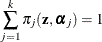# The HPFMM Procedure

### Notation for the Finite Mixture Model

Subsections:

The general expression for the finite mixture model fitted with the HPFMM procedure is as follows: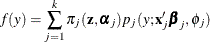The number of components in the mixture is denoted as k. The mixture probabilities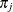can depend on regressor variablesand parameters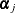. By default, the HPFMM procedure models these probabilities using a logit transform if k = 2 and as a generalized logit model if k > 2. The component distributions
can also depend on regressor variables in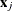, regression parameters
, and possibly scale parameters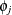. Notice that the component distributionsare indexed by j since the distributions might belong to different families. For example, in a two-component model, you might model one component as a normal (Gaussian) variable and the second component as a variable with a t distribution with low degrees of freedom to manage overdispersion.

The mixture probabilitiessatisfy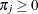, for all j, and